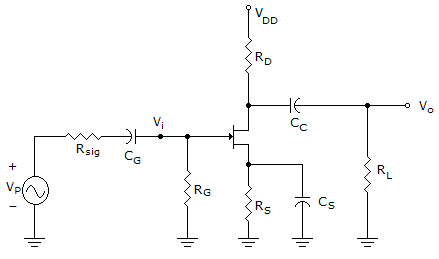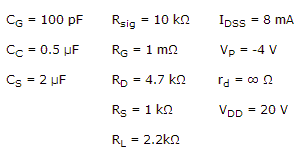# Electronic Devices - BJT and FET Frequency Response - Discussion

### Discussion :: BJT and FET Frequency Response - General Questions (Q.No.4)

4.

Determine the lower cutoff frequency of this network.[A]. 15.8 Hz [B]. 46.13 Hz [C]. 238.73 Hz [D]. 1575.8 Hz

Explanation:

No answer description available for this question.

 Pulls said: (Oct 16, 2014) f = 1/2*pi*(rsi+rg)*cg formula can be used.

 Lily said: (Apr 5, 2018) RG should be 1M ohms.

 Kweku said: (May 29, 2018) @Pulls. The formula gives D as answer when RG is taken as 1M ohms.

 Paul said: (Jul 7, 2018) f=1/2π*Req*Cs. where: Req= Rs II 1/gm. Ans. 238.73.

 Paul said: (Jul 7, 2018) The cut off frequency at Cs. f=1/2π*(Rs II 1/gm)*Cs.

 John said: (Sep 20, 2018) What's the value of gm?

 Erwin John said: (Aug 29, 2019) @John. For gm, get the value of current Id first. Use the formula: Id = Idss (1- (Vgs/Vp))^2 but replace Vgs with -Id(Rs). Then you will get two values of Id. Choose the lower value which is equal to 2mA. The lower value of Id means that the FET is operating below the zero bias current, Idss. Get Vgs using the formula Vgs = -Id(Rs). And use this formula to get gm. gm = (2Idss/|Vp|)(1-(Vgs/Vp)).

 Abdul Haseeb said: (Sep 19, 2019) How to calculate gm, Vgs, Id? Explain.

 Abdul Haseeb said: (Sep 19, 2019) How to calculate voltage gain, z input impedance and output impedance?